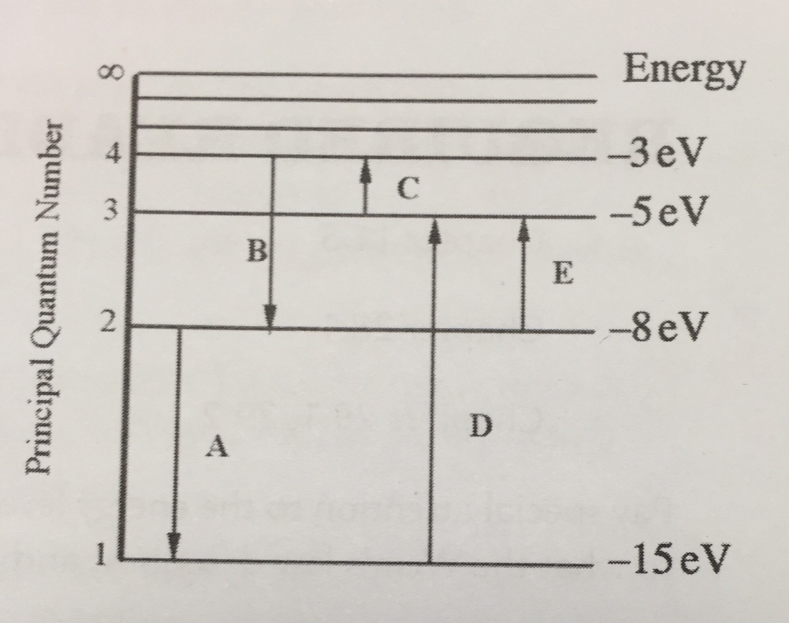# Problem: For this question consider the figure to the right shows an energy level diagram for a certain atom (not hydrogen). Several transitions are shown and are labeled by letters. a.) Which transition corresponds to the absorption of the photon with the longest wavelength? b.) Which transition corresponds to the absorption of the photon with the shortest wavelength?

###### FREE Expert Solution

Emission occurs only when electrons get transferred from the outer orbit to the inner one (higher energy level to lower energy level).

Absorption is when electron moves from inner orbit to outer orbit.

The transition from 4 to 2 and 2 to 1 is emission.

On the other hand, the transition from 1 to 3 or 2 to 3 or 3 to 4 is absorption

a)

A large energy difference between orbits implies that the frequency is large and the wavelength is shorter.

99% (410 ratings)###### Problem Details

For this question consider the figure to the right shows an energy level diagram for a certain atom (not hydrogen). Several transitions are shown and are labeled by letters.a.) Which transition corresponds to the absorption of the photon with the longest wavelength?

b.) Which transition corresponds to the absorption of the photon with the shortest wavelength?

Frequently Asked Questions

What scientific concept do you need to know in order to solve this problem?

Our tutors have indicated that to solve this problem you will need to apply the Nuclear Physics concept. You can view video lessons to learn Nuclear Physics. Or if you need more Nuclear Physics practice, you can also practice Nuclear Physics practice problems.